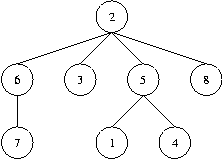Online JudgeProblem SetAuthorsOnline ContestsUser
Web Board
F.A.Qs
Statistical Charts
Problems
Submit Problem
Online Status
Prob.ID:
Register
Authors ranklist
Current Contest
Past Contests
Scheduled Contests
Award Contest
Register
Language:
Code the Tree
 Time Limit: 1000MS Memory Limit: 65536K Total Submissions: 3036 Accepted: 1179

Description

A tree (i.e. a connected graph without cycles) with vertices numbered by the integers 1, 2, ..., n is given. The "Prufer" code of such a tree is built as follows: the leaf (a vertex that is incident to only one edge) with the minimal number is taken. This leaf, together with its incident edge is removed from the graph, while the number of the vertex that was adjacent to the leaf is written down. In the obtained graph, this procedure is repeated, until there is only one vertex left (which, by the way, always has number n). The written down sequence of n-1 numbers is called the Prufer code of the tree.
Your task is, given a tree, to compute its Prufer code. The tree is denoted by a word of the language specified by the following grammar:
```T ::= "(" N S ")"
S ::= " " T S
| empty
N ::= number```

That is, trees have parentheses around them, and a number denoting the identifier of the root vertex, followed by arbitrarily many (maybe none) subtrees separated by a single space character. As an example, take a look at the tree in the figure below which is denoted in the first line of the sample input. To generate further sample input, you may use your solution to Problem 2568.
Note that, according to the definition given above, the root of a tree may be a leaf as well. It is only for the ease of denotation that we designate some vertex to be the root. Usually, what we are dealing here with is called an "unrooted tree".

Input

The input contains several test cases. Each test case specifies a tree as described above on one line of the input file. Input is terminated by EOF. You may assume that 1<=n<=50.

Output

For each test case generate a single line containing the Prufer code of the specified tree. Separate numbers by a single space. Do not print any spaces at the end of the line.Sample Input

```(2 (6 (7)) (3) (5 (1) (4)) (8))
(1 (2 (3)))
(6 (1 (4)) (2 (3) (5)))
```

Sample Output

```5 2 5 2 6 2 8
2 3
2 1 6 2 6
```

Source

[Submit]   [Go Back]   [Status]   [Discuss]Home PageGo BackTo top